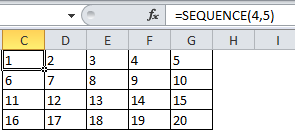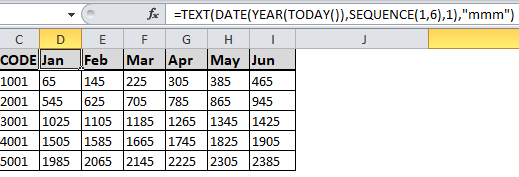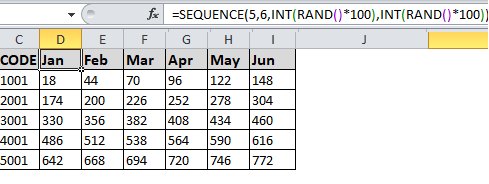## Excel Office

Excel How Tos, Tutorials, Tips & Tricks, Shortcuts

# SEQUENCE function: Description, Usage, Syntax, Examples and Explanation

## What is SEQUENCE function in Excel?

SEQUENCE function is one of the Math and Trig functions in Microsoft Excel that allows you to generate a list of sequential numbers in an array, such as 1, 2, 3, 4.

## Syntax of SEQUENCE function

=SEQUENCE(rows, [columns], [start], [step])

## Example of SEQUENCE function

Example 1

In the following example, we created an array that’s 4 rows tall by 5 columns wide with =SEQUENCE(4,5).Example 2

If you need to create a quick sample dataset, here’s an example using SEQUENCE with TEXT, DATE, YEAR, and TODAY to create a dynamic list of months for a header row, where the underlying date will always be the current year. Our formula is: =TEXT(DATE(YEAR(TODAY()),SEQUENCE(1,6),1),”mmm”).Example 3

Here’s an example of nesting SEQUENCE with INT and RAND to create a 5 row by 6 column array with a random set of increasing integers. Our formula is: =SEQUENCE(5,6,INT(RAND()*100),INT(RAND()*100)).In addition, you could use =SEQUENCE(5,1,1001,1000) to create the sequential list of GL Code numbers in the examples.